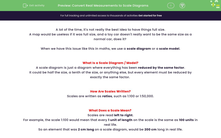# Convert Real Measurements to Scale Diagrams

In this worksheet, students will apply scale factors to find the scale values of specific, real life elements or calculate the scale which has been used to create a scale diagram or model.Key stage:  KS 4

Year:  GCSE

GCSE Subjects:   Maths

GCSE Boards:   AQA, Eduqas, Pearson Edexcel, OCR,

Curriculum topic:   Ratio, Proportion and Rates of Change, Mensuration

Curriculum subtopic:   Ratio, Proportion and Rates of Change Units and Measurement

Difficulty level:#### Worksheet Overview

A lot of the time, it's not really the best idea to have things full size.

A map would be useless if it was full size, and a toy car doesn't really want to be the same size as a normal car, does it?

When we have this issue like this in maths, we use a scale diagram or a scale model.

What Is a Scale Diagram / Model?

A scale diagram is just a diagram where everything has been reduced by the same factor.

It could be half the size, a tenth of the size, or anything else, but every element must be reduced by exactly the same factor.

How Are Scales Written?

Scales are written as ratios, such as 1:100 or 1:50,000.

What Does a Scale Mean?

Scales are read left to right.

For example, the scale 1:100 would mean that every 1 unit of length on the scale is the same as 100 units in real life.

So an element that was 2 cm long on a scale diagram, would be 200 cm long in real life.

Let's look at this concept in action with some examples now.

e.g. A real car is 300 cm tall. A model of the car is created using a scale of 1:100. How tall would the model car be?

The scale tells us that every 1 cm on the model car will represent 100 cm in real life.

So all we need to do is to divide the real height by the scale factor, to find the height of the model:

300 ÷ 100 = 3 cm

So the height of the model car is 3 cm.

e.g. A model is made of a 2 m tall man. If the model is 4 cm tall, what scale has been used?

The first thing we should notice here is that the units used are different, so we need to make them the same before we start:

There are 100 cm in 1 m, so 2 m = 200 cm.

Now, we need to write these numbers as a ratio (remember that the model comes first):

4:200

Our final step is to simplify this ratio:

4:200 ÷ 4

1:50

In this activity, we will apply scale factors to find the scale values of specific, real life elements or calculate the scale which has been used to create a scale diagram or model.

### What is EdPlace?

We're your National Curriculum aligned online education content provider helping each child succeed in English, maths and science from year 1 to GCSE. With an EdPlace account you’ll be able to track and measure progress, helping each child achieve their best. We build confidence and attainment by personalising each child’s learning at a level that suits them.

Get started